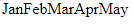# JavaScript Iterators and Iterables

## JavaScript Iterators and Iterables

In this article, I am going to discuss JavaScript Iterators and Iterables with Examples. Please read our previous article where we discussed JavaScript yield Keyword in detail. As part of this article, you will learn the following pointers in detail.

1. Iterator – Observer Interface
2. Iterables and its Properties
3. Why iterable were added in ES6?
4. Built-in Iterables
6. Maps and Sets
7. String is iterable
8. Iterable Protocolfor…of loop
##### JavaScript Iterators and Iterables:

A generator object is both iterator and iterable. An iterable is a data structure that wants to make its elements accessible to the public. It does so by implementing a method whose key is Symbol.iterator. That method is a factory for iterators. That is, it will create iterators. An iterator is a pointer for traversing the elements of a data structure.

##### Iterator – Observer Interface

A generator is a combination of two things – an Iterator and an Observer

##### Iterator

It’s an Object that allows us to access items from a list or collection one at a time while keeping track of its current position within that sequence.

An object that is returned by the iterable interface is also an iterator object. A generator(iterator) is a producer. In iteration the consumer PULLs the value from the producer.

An iterator is something when invoked returns an iterable. An iterable is something we can iterate upon. From ES6/ES2015 onwards, all collections (Array, Map, Set, WeakMap, WeakSet) all are built-in Iterables.

##### Example:
```<html>
<title>JavaScript Iterator Example</title>
<body>
<script>
function* gen()
{
yield 5;
yield 6;
}
let a = gen();
console.log(a.next());
console.log(a.next());
console.log(a.next());
</script>
</body>
</html>```

Output: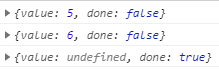Whenever we call next(), we’re essentially pull-ing value from the Iterator and pause the execution at yield. This method returns an object with two properties: done and value and when next() calls reach to the end of sequence then the done property set to true else remains false .

The next time we call next(), the execution resumes from the previously paused state. The next() method yields the value, where done=true means that the iteration is finished, otherwise the value is the next value. and the next on iterator return is:

{value: ‘Current value of iteration’, done: ‘true/false’}

##### Observer

A generator is also an observer using which we can send some values back into the generator.

Example:

```<html>
<title>JavaScript Observer Example</title>
<body>
<script>
function* gen() {
document.write('<br>observer:', yield 1);
}
var a = gen();
var i = a.next();
while (!i.done) {
document.write('<br>iterator:', i.value);
i = a.next(100);
}
</script>
</body>
</html>```

Output: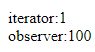Here we can see that yield 1 is used like an expression that evaluates to some value. The value it evaluates to is the value sent as an argument to the a.next() function call. So, for the first time i.value will be the first value yielded (1), and when we continuing the iteration to the next state, we send a value back to the generator using a.next(100).

##### Iterables

A generator is iterable. It can be looped over with a forof statement, and used in other constructs that depend on the iteration protocol. The iterable is an interface that specifies that an object can be accessible if it implements a method whose key is [symbol.iterator].

##### Iterables Properties:
• Objects that are used in for…of are called iterable
• Iterables must implement the method name Symbol.iterator
• The result of obj[Symbol.iterator] is called an iterator. that takes care of the further iteration process.
• A iterator must have next() method, which return an object {done:Boolean,value:true\false}

where done=true signifies the end of iteration execution else the value will contain the next value in the iteration.

• the Symbol.iterator is automatically called by for…of loop
• some built-in iterable such as string,array have Symbol.iterator method.
##### Why iterable were added in ES6?

Without iterable, it is difficult to manage the iteration on data for various types of data structures i.e. iterating on an array is different from iterating on an object.

Also, ES6 has introduced new data structures like sets and maps so it will become more complicated to write logic as per data structure for iterations. This is where the iterable interface came into picture.

There are two things to observe here

1. Data consumer — how the iteration takes place like using loops, spread operator, array.from method, destructuring via an array pattern, etc.
2. Data source — what data structure we choose like an array, maps, string, etc. to iterate on.
##### Built-in Iterables

Some of the built-in iterables are given below, because their prototype objects all have the Symbol.iterator method.

• Arrays and TypeArray
• Strings — iterate over each character
• Maps — iterate over its key-value pairs
• Sets — iterate over its element etc.
• arguments —An array- like variable in a function

Some other constructs in JS that use iterables are

for-of loop :The for-of loops require an iterable. Otherwise, it will throw a TypeError.

function* range(n) {
for (let i = 0; i < n; ++i) {
yield i;
}
}

// looping
for (let n of range(10)) {
// n takes on the values 0, 1, … 9
}

To make the range iterable and to make for..of work. we have to add a method named Symbol.iterator (a special built-in symbol just for that) to the object.

1. When for..of starts, it calls and execute that method once or errors if not found. The method must return an iterator – an object with the method next.
2. for..of works only with the returned object.
3. When for..of wants the next value, it calls next() on that object.
4. The result of next() is in the form {done: Boolean, value: any}, where done=true means that the iteration execution is finished, otherwise the value is the next value.
##### Example:
```<html>
<title>JavaScript range[Symbol.iterator] array Example</title>
<body>
<script>
let range = {
from: 1,
to: 5
};

// 1. call to for..of initially calls this
range[Symbol.iterator] = function () {

// ...it returns the iterator object with the method next():
// 2. Onward, for..of works only with this iterator, asking it for next values
return {
current: this.from,
last: this.to,

// 3. next() is called on each iteration by the for..of loop
next() {
// 4. it should return the value as an object {done: Boolean, value: any}
if (this.current <= this.last) {
return { done: false, value: this.current++ };
} else {
return { done: true };
}
}
};
};

// now it works!
for (let num of range) {
document.write('<br>',num); // 1, then 2, 3, 4, 5
}
</script>
</body>
</html>```

Output: 1 2 3 4 5

One thing we need to note here is that:

• The range itself does not have the next() method.
• In place of, another object called “iterator” is created by the call to the range[Symbol.iterator](), and its next() method generates values for the iteration.
• Destructuring of Arrays — Destructuring happens due to iterables
##### The code:

const array = [‘Mon’, ‘Tue’, ‘Wed’, ‘Thu’, ‘Fri’];
const [first, , third, , last] = array;

is equal to:

```<html>
<title>JavaScript Destructuring of array Example</title>
<body>
<script>
const array = ['Mon', 'Tue', 'Wed', 'Thu', 'Fri'];
const iterator = array[Symbol.iterator]();
const first = iterator.next().value
document.write('first: ', first);
iterator.next().value // Since it was skipped, so it's not assigned
const third = iterator.next().value
document.write('<br>third: ', third);
iterator.next().value // Since it was skipped, so it's not assigned
const last = iterator.next().value
document.write('<br>last: ', last);
</script>
</body>
</html>```

Output: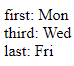what is spread operator will discuss more into the Spread chapter as of now let focus on how the spread operator code can be written to be iterable.

The code

const array = [‘Mon’, ‘Tue’, ‘Wed’, ‘Thu’, ‘Fri’];
const newArray = [1, …array, 2, 3]

Is equal to

```<html>
<body>
<script>
const array = ['Mon', 'Tue', 'Wed', 'Thu', 'Fri'];
const iterator = array[Symbol.iterator]();
const newArray = ;
for (let nextValue = iterator.next(); nextValue.done !== true; nextValue = iterator.next()) {
newArray.push(nextValue.value);
}
newArray.push(2)
newArray.push(3)
document.write('new Array= ', newArray);
</script>
</body>
</html>```

Output: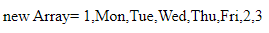##### Maps:

what is Map will discuss more into the Map chapter topic as of now let focus on how the Map code can be written to be iterable. The constructor of a Map turns iterable over [key, value] pairs into a Map

```<html>
<title>JavaScript Map Example</title>
<body>
<script>
const map = new Map([[1, 'one'], [2, 'two']]);
map.get(1)

document.write('Map= ', map.get(1));// one
</script>
</body>
</html>```

Output: Map= one

##### Sets:

What is Sets operator will discuss more into Set chapter as of now let focus on how the Sets code can be written to be iterable. The constructor of a Set turns an iterable over elements into a Set

```<html>
<title>JavaScript Sets Example</title>
<body>
<script>
const set = new Set(['a', 'b', 'c']);
set.has('c');

document.write('Set= ', set.has('c'));// true
</script>
</body>
</html>```

Output: Set= true

##### String is iterable

Arrays and strings are the most widely used common built-in iterables. For a string for…of loop iterate over its characters

##### Example1:
```<html>
<title>JavaScript string iterable Example1</title>
<body>
<script>
for (let char of "JavaScript") {
// triggers 10 times: once for each character
document.write(char); // J, then a, then v, then a,S,c,r,i,p,t
}
</script>
</body>
</html>```

Output: JavaScript

##### Example2:
```<html>
<title>JavaScript string iterable Example2</title>
<body>
<script>
let str = 'a😂b';
for (let char of str) {
document.write('<br>', char); // a, and then 😂 and then b
}
</script>
</body>
</html>```

Output: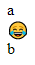So, the string iterator will look like

##### Example string iterator:
```<html>
<title>JavaScript string iterator Example</title>
<body>
<script>
let str = "Hello JavaScript";

// does the same as
// for (let char of str) alert(char);

let iterator = str[Symbol.iterator]();

while (true) {
let result = iterator.next();
if (result.done) break;
document.write(' ', result.value); // outputs characters one by one
}
</script>
</body>
</html>```

Output: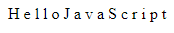the iterator gives us more control over the data structure like string than for…of. Thus, we can split the iteration, stop its execution then later we can resume it. So, we can conclude that the iterable are objects that implement the Symbol.iteraor method.

##### Array-likes

Array-like objects that are iterable that have length and indexes which make them look alike arrays. array-likes are not usually arrays they don’t have push, pop method like an array does. The object that is array-like is not iterable. If we try to iterate over it, we will receive the TypeError.

##### Example:
```<html>
<title>JavaScript array-like Example</title>
<body>
<script>
let arrayLike = { // has indexes and length => array-like
0: "Hello",
1: "World",
length: 2
};

// Error (no Symbol.iterator)
for (let item of arrayLike) {
document.write(' ', item);//TypeError :arraylike is not iterable
}
</script>
</body>
</html>```

Output: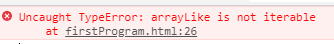##### Array.from

Array.from is a global method that takes iterable or array-like object value and then converts them into the original array. On which we can perform the normal array method such as push, pop.

Syntax: Array.from(arrayLike, mapfn, [thisArg])

Creates an array from an iterable object.

##### arrayLike:

An array-like object to convert to an array. The second optional argument mapfn is a function that will be applied to each element of the array and thisArg allow us to set this for it.

##### Example:
```<html>
<title>JavaScript Array.from Example</title>
<body>
<script>
let arrayLike = {
0: "Hello",
1: "JavaScript",
2: "array-like",
length: 3
};

let arr = Array.from(arrayLike); // takes the arraylike object
</script>
</body>
</html>```

Output: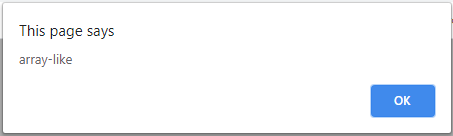We can use Array.from to convert the string into an array of characters.

##### Example-1 Array.from to convert string to array of characters:
```<html>
<title>JavaScript Array.from to convert string to array of characters Example-1</title>
<body>
<script>
let str = 'ab😂cd';

// splits str into array of characters
let chars = Array.from(str);

document.write(' ', chars); // a
document.write(' ', chars); // b
document.write(' ', chars); // 😂
document.write(' ', chars); // c
document.write(' ', chars); // d
document.write(' length= ', chars.length); // 5
</script>
</body>
</html>```

Output: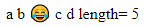##### Example-2:
```<html>
<title>JavaScript Array.from to convert string to array of characters Example-2</title>
<body>
<script>
let str = 'ab😂cd';

let chars = []; // Array.from internally does the same loop
for (let char of str) {
chars.push(char);
}

document.write(chars);
</script>
</body>
</html>```

Output: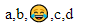##### array[Symbol.iterator]:

Example:

```<html>
<title>JavaScript array[Symbol.iterator] example</title>
<body>
<script>
const array = ['Jan', 'Feb', 'Mar'];

const it = array[Symbol.iterator]();

// and on this iterator method we have ‘next’ method

document.write(JSON.stringify(it.next()));
//{ value: "Jan", done: false }

document.write('<br>', JSON.stringify(it.next()));
//{ value: "Feb", done: false }

document.write('<br>', JSON.stringify(it.next()));
//{ value: "Mar", done: false }

document.write('<br>', JSON.stringify(it.next()));
/* Actual it.next() will be { value: undefined,
done: true } but here you will get
{done: true} output because of JSON.stringify
as it omits undefined values*/
</script>
</body>
</html>```

Output: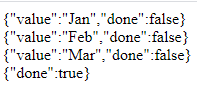##### Iterable Protocol:

The Iterable protocol we already discussed in the earlier section let us walkthrough over it once again.

The object must have Symbol.iterator key which in turn returns the object which itself follows the iterator protocol. The object must define the next() method which returns the iteratorResult Object with two properties value and done. value contains the actual value from the iteration and done contains the Boolean value indicating whether the iteration process execution finished or not by having value as true/false.

Syntax: {value: ‘item value’, done: true / false}

##### Example:
```<html>
<title>JavaScript iterable protocol example</title>
<body>
<script>
var iterableObj = {
i: 0,
[Symbol.iterator]() {
var that = this;
return {
next() {
if (that.i < 5) {
return { value: that.i++, done: false }
} else {
return { value: undefined, done: true }
}
}
}
}
}
for (let itemValue of iterableObj) { document.write(itemValue) }
</script>
</body>
</html>```

Output: 01234

##### for…of loop:

Using for…of loop, we can iterate over any object which follows the iterable protocol which we discussed in the previous section. The for…of loop is going to take out the value that gets a return by calling the next() method each time.

##### Example:
```<html>
<title>JavaScript for…of loop example</title>
<body>
<script>
const array = ['Jan', 'Feb', 'Mar', 'Apr', 'May'];

const arrIterator = array[Symbol.iterator]();

for (let value of arrIterator) { document.write(value) }
</script>
</body>
</html>```

Output: